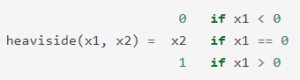Related Articles

# Python | Numpy np.heaviside() method

• Last Updated : 02 Dec, 2019

With the help of `np.heaviside()` method, we can get the heaviside step function by using `np.heaviside()` method.Syntax : `np.heaviside(array1, array2 or value)`
Return : Return the heaviside series.

Example #1 :
In this example we can see that by using `np.heaviside()` method, we are able to get the array of series of heaviside step function by using this method.

 `# import numpy``import` `numpy as np`` ` `x ``=` `np.array([``-``1.5``, ``0.5``, ``0``, ``0.5``, ``1.5``])``# using np.heaviside() method``gfg ``=` `np.heaviside(x, ``0.5``)`` ` `print``(gfg)`

Output :

[0. 1. 0.5 1. 1.]

Example #2 :

 `# import numpy``import` `numpy as np`` ` `x ``=` `np.zeros(``5``)``y ``=` `np.array([``-``1.5``, ``0.5``, ``0``, ``0.5``, ``-``1.5``])`` ` `# using np.heaviside() method``gfg ``=` `np.heaviside(x, y)`` ` `print``(gfg)`

Output :

[-1.5 0.5 0. 0.5 -1.5]

Attention geek! Strengthen your foundations with the Python Programming Foundation Course and learn the basics.

To begin with, your interview preparations Enhance your Data Structures concepts with the Python DS Course. And to begin with your Machine Learning Journey, join the Machine Learning – Basic Level Course

My Personal Notes arrow_drop_up